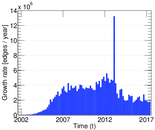# Wikipedia edits (nl)

This is the bipartite edit network of the Dutch Wikipedia. It contains users and pages from the Dutch Wikipedia, connected by edit events. Each edge represents an edit. The dataset includes the timestamp of each edit.

 Code `nl` Internal name `edit-nlwiki` Name Wikipedia edits (nl) Data source http://dumps.wikimedia.org/ AvailabilityDataset is available for download Consistency checkDataset passed all tests Category Authorship network Dataset timestamp 2017-10-20 Node meaning User, article Edge meaning Edit Network formatBipartite, undirected Edge typeUnweighted, multiple edges Temporal dataEdges are annotated with timestamps

## Statistics

 Size n = 4,021,196 Left size n1 = 220,847 Right size n2 = 3,800,349 Volume m = 41,592,827 Unique edge count m̿ = 22,142,951 Wedge count s = 1,940,630,768,592 Cross count x = 6.664 47 × 1022 Maximum degree dmax = 1,438,739 Maximum left degree d1max = 1,438,739 Maximum right degree d2max = 206,680 Average degree d = 20.686 8 Average left degree d1 = 188.333 Average right degree d2 = 10.944 5 Average edge multiplicity m̃ = 1.878 38 Size of LCC N = 3,997,990 Diameter δ = 11 50-Percentile effective diameter δ0.5 = 3.438 08 90-Percentile effective diameter δ0.9 = 3.959 22 Median distance δM = 4 Mean distance δm = 3.788 03 Gini coefficient G = 0.867 044 Balanced inequality ratio P = 0.142 122 Left balanced inequality ratio P1 = 0.034 984 5 Right balanced inequality ratio P2 = 0.203 568 Relative edge distribution entropy Her = 0.726 919 Tail power law exponent γt = 2.761 00 Degree assortativity ρ = −0.136 245 Degree assortativity p-value pρ = 0.000 00

## Plots

### Degree distribution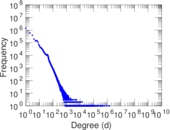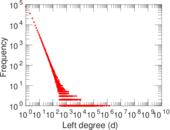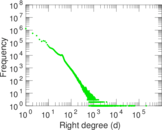### Cumulative degree distribution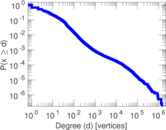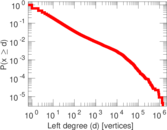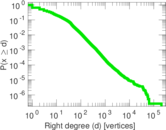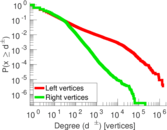### Spectral distribution of the adjacency matrix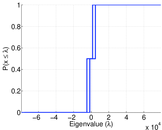### Spectral distribution of the normalized adjacency matrix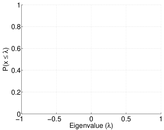### Spectral distribution of the Laplacian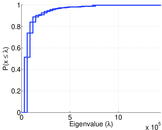### Spectral graph drawing based on the adjacency matrix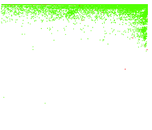### Spectral graph drawing based on the Laplacian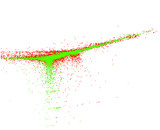### Spectral graph drawing based on the normalized adjacency matrix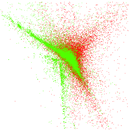### Zipf plot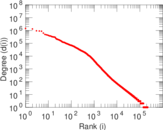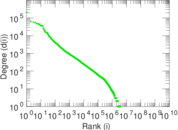### Hop distribution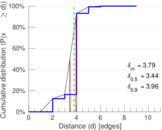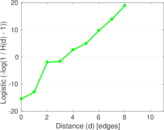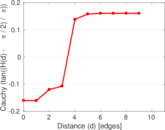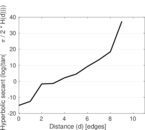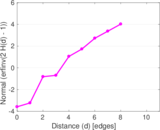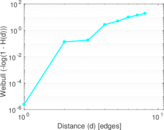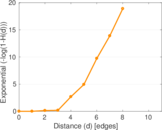### Edge weight/multiplicity distribution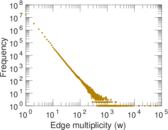### Temporal distribution